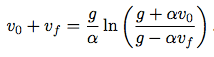# Proving an inequality from an equation

• I
Happiness
Given that ##v_0## and ##v_f## are positive variables related by the equationwhere ##g## and ##\alpha## are positive constants.

Can you show that ##v_f<v_0## for all positive values of ##v_0## using a non-graphical method?

Physically, ##v_0## and ##v_f## are the initial and final speeds (at the same height) of a ball thrown upwards in a medium with a drag force ##F=-m\alpha v##.

Mentor
Yes. Hint: -ln(1-x) > ln(1+x) for positive x.

Happiness
Yes. Hint: -ln(1-x) > ln(1+x) for positive x.

But this is only true for positive ##x<1##. How then can we show ##v_f<v_0## for all positive values of ##v_0##?

Using the hint, I can only get to ##y-\ln(1+y)>\ln(1+x)-x##, where ##x=\frac{\alpha v_0}{g}## and ##y=\frac{\alpha v_f}{g}##.

Mentor
But this is only true for positive ##x<1##.
Your logarithm is not defined otherwise, a vf where the logarithm is not defined cannot be a solution.

But does that have a solution at all?
Let ##c=\frac{g}{\alpha}##, and using the inequality for the denominator, I get

$$v_0 + v_f \leq c \ln(1+v_0/c) + c \ln(1+v_f/c)$$
That is wrong, as x > ln(1+x).

Happiness
But does that have a solution at all?
Let ##c=\frac{g}{\alpha}##, and using the inequality for the denominator, I get

$$v_0 + v_f \leq c \ln(1+v_0/c) + c \ln(1+v_f/c)$$
That is wrong, as x > ln(1+x).

Using the hint

##c\ln(\frac{1+v_0/c}{1-v_f/c})>c\ln(1+v_0/c)+c\ln(1+v_f/c)##

So

##v_0+v_f>c\ln(1+v_0/c)+c\ln(1+v_f/c)##

Your logarithm is not defined otherwise, a vf where the logarithm is not defined cannot be a solution.

Indeed, ##v_f<\frac{g}{\alpha}##. It can be easily shown that ##\frac{g}{\alpha}## is the terminal velocity. So, indeed, only positive ##x<1## needs to be considered.

Mentor
Oh right, wrong direction. Hmm, making it symmetric does not help. Okay, more mathematics.

Introduce V as ##V=\frac{v_0 \alpha}{g}## and ##a>0## as ##v_f=a v_0##. Then we get
$$V+aV = ln(1+V) - ln(1-aV)$$
Here is a plot

Consider f(x)=x-ln(1+x) where x>0.
##f'(x)=\frac{x}{x+1} > 0##
Rewrite the equation from above:
$$V-ln(1+V)- aV + ln(1-aV) = 2aV$$
$$f(V) - f(aV) = 2 aV$$As a>0, V>0, we get
$$f(V) > f(aV)$$
We established that f is increasing monotonically, therefore a<1 which corresponds to ##v_f < v_0##.

Happiness
Rewrite the equation from above:
$$V-ln(1+V)- aV + ln(1-aV) = 2aV$$
$$f(V) - f(aV) = 2 aV$$

This doesn't seem right.

##f(V) - f(aV)=V-\ln(1+V)-\bigg(aV - \ln(1+aV)\bigg)=V-\ln(1+V)- aV + \ln(1+aV)##

The last term is different from the LHS in the previous step.

And the RHS should be ##-2aV##.

Last edited:
Mentor
That should work now:
Consider f(x)=x-ln(1+x) where x>-1.
$$f'(x)=\frac{x}{x+1}$$
Note that |f'(-x)| > |f'(x)| for 0<x<1.
Via integration from 0 to x, we can conclude that f(-x)>f(x) for positive x.
$$V+aV = ln(1+V) - ln(1-aV)$$
$$V- ln(1+V) = -aV - ln(1+(-aV))$$
$$f(V) = f(-aV) > f(aV)$$
$$f(V) > f(aV)$$
The function f has a minimum at x=0 and is monotonically increasing for positive x.
=> a < 1

•Happiness